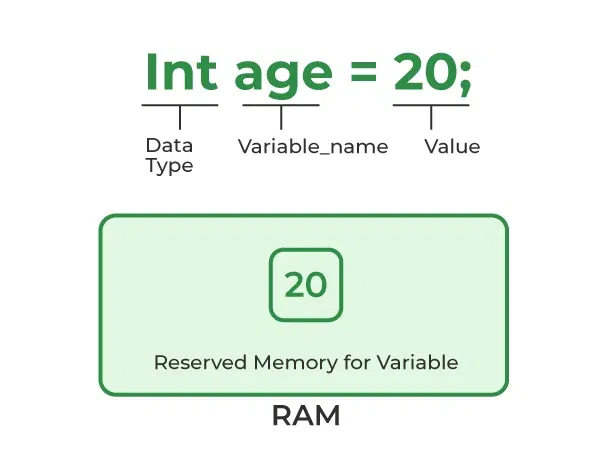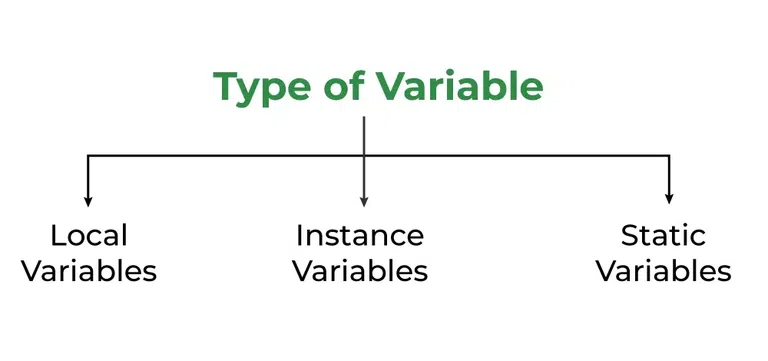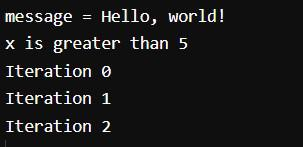GFG App
Open AppBrowser
Continue

# Java Variables

In Java, Variables are the data containers that save the data values during Java program execution. Every Variable in Java is assigned a data type that designates the type and quantity of value it can hold. A variable is a memory location name for the data.

## Variables in Java

Java variable is a name given to a memory location. It is the basic unit of storage in a program.

• The value stored in a variable can be changed during program execution.
• Variables in Java are only a name given to a memory location. All the operations done on the variable affect that memory location.
• In Java, all variables must be declared before use.

## How to Declare Variables in Java?

We can declare variables in Java as pictorially depicted below as a visual aid.From the image, it can be easily perceived that while declaring a variable, we need to take care of two things that are:

1. datatype: Type of data that can be stored in this variable.
2. data_name: Name was given to the variable.

In this way, a name can only be given to a memory location. It can be assigned values in two ways:

• Variable Initialization
• Assigning value by taking input

## How to Initialize Variables in Java?

It can be perceived with the help of 3 components that are as follows:

• datatype: Type of data that can be stored in this variable.
• variable_name: Name given to the variable.
• value: It is the initial value stored in the variable.Illustrations:

```// Declaring float variable
float simpleInterest;

// Declaring and initializing integer variable
int time = 10, speed = 20;

// Declaring and initializing character variable
char var = 'h'; ```

## Types of Variables in Java

Now let us discuss different types of variables  which are listed as follows:

1. Local Variables
2. Instance Variables
3. Static VariablesLet us discuss the traits of every type of variable listed here in detail.

### 1. Local Variables

A variable defined within a block or method or constructor is called a local variable.

• These variables are created when the block is entered, or the function is called and destroyed after exiting from the block or when the call returns from the function.
• The scope of these variables exists only within the block in which the variables are declared, i.e., we can access these variables only within that block.
• Initialization of the local variable is mandatory before using it in the defined scope.

#### Time Complexity of the Method:

```Time Complexity: O(1)
Auxiliary Space: O(1)```

Below is the implementation of the above approach:

## Java

 `// Java Program to implement` `// Local Variables` `import` `java.io.*;`   `class` `GFG {` `    ``public` `static` `void` `main(String[] args)` `    ``{` `        ``// Declared a Local Variable` `        ``int` `var = ``10``;`   `        ``// This variable is local to this main method only` `        ``System.out.println(``"Local Variable: "` `+ var);` `    ``}` `}`

Output

`Local Variable: 10`

Example :

## Java

 `package` `a;` `public` `class` `LocalVariable {` `    `  `    ``public` `static` `void` `main(String[] args) {` `        ``int` `x = ``10``; ``// x is a local variable` `        ``String message = ``"Hello, world!"``; ``// message is also a local variable` `        `  `        ``System.out.println(``"x = "` `+ x);` `        ``System.out.println(``"message = "` `+ message);` `        `  `        ``if` `(x > ``5``) {` `            ``String result = ``"x is greater than 5"``; ``// result is a local variable` `            ``System.out.println(result);` `        ``}` `        `  `        ``// Uncommenting the line below will result in a compile-time error` `        ``// System.out.println(result);` `        `  `        ``for` `(``int` `i = ``0``; i < ``3``; i++) {` `            ``String loopMessage = ``"Iteration "` `+ i; ``// loopMessage is a local variable` `            ``System.out.println(loopMessage);` `        ``}` `        `  `        ``// Uncommenting the line below will result in a compile-time error` `        ``// System.out.println(loopMessage);` `    ``}` `}`

output :### 2. Instance Variables

Instance variables are non-static variables and are declared in a class outside of any method, constructor, or block.

• As instance variables are declared in a class, these variables are created when an object of the class is created and destroyed when the object is destroyed.
• Unlike local variables, we may use access specifiers for instance variables. If we do not specify any access specifier, then the default access specifier will be used.
• Initialization of an instance variable is not mandatory. Its default value is dependent on the data type of variable. For String it is null, for float it is 0.0f, for int it is 0, for Wrapper classes like Integer it is null, etc.
• Instance variables can be accessed only by creating objects.
• We initialize instance variables using constructors while creating an object. We can also use instance blocks to initialize the instance variables.

#### The complexity of the method:

```Time Complexity: O(1)
Auxiliary Space: O(1)```

Below is the implementation of the above approach:

## Java

 `// Java Program to demonstrate` `// Instance Variables` `import` `java.io.*;`   `class` `GFG {`   `    ``// Declared Instance Variable` `    ``public` `String geek;` `    ``public` `int` `i;` `    ``public` `Integer I;` `    ``public` `GFG()` `    ``{` `        ``// Default Constructor` `        ``// initializing Instance Variable` `        ``this``.geek = ``"Shubham Jain"``;` `    ``}`   `    ``// Main Method` `    ``public` `static` `void` `main(String[] args)` `    ``{` `        ``// Object Creation` `        ``GFG name = ``new` `GFG();`   `        ``// Displaying O/P` `        ``System.out.println(``"Geek name is: "` `+ name.geek);` `        ``System.out.println(``"Default value for int is "` `                           ``+ name.i);` `      `  `        ``// toString() called internally` `        ``System.out.println(``"Default value for Integer is "` `                           ``+ name.I);` `    ``}` `}`

Output

```Geek name is: Shubham Jain
Default value for int is 0
Default value for Integer is null```

### 3. Static Variables

Static variables are also known as class variables.

• These variables are declared similarly to instance variables. The difference is that static variables are declared using the static keyword within a class outside of any method, constructor, or block.
• Unlike instance variables, we can only have one copy of a static variable per class, irrespective of how many objects we create.
• Static variables are created at the start of program execution and destroyed automatically when execution ends.
• Initialization of a static variable is not mandatory. Its default value is dependent on the data type of variable. For String it is null, for float it is 0.0f, for int it is 0, for Wrapper classes like Integer it is null, etc.
• If we access a static variable like an instance variable (through an object), the compiler will show a warning message, which won’t halt the program. The compiler will replace the object name with the class name automatically.
• If we access a static variable without the class name, the compiler will automatically append the class name. But for accessing the static variable of a different class, we must mention the class name as 2 different classes might have a static variable with the same name.
• Static variables cannot be declared locally inside an instance method.
• Static blocks can be used to initialize static variables.

#### The complexity of the method:

```Time Complexity: O(1)
Auxiliary Space: O(1)```

Below is the implementation of the above approach:

## Java

 `// Java Program to demonstrate` `// Static variables` `import` `java.io.*;`   `class` `GFG {` `    ``// Declared static variable` `    ``public` `static` `String geek = ``"Shubham Jain"``;` `  `  `    ``public` `static` `void` `main(String[] args)` `    ``{`   `        ``// geek variable can be accessed without object` `        ``// creation Displaying O/P GFG.geek --> using the` `        ``// static variable` `        ``System.out.println(``"Geek Name is : "` `+ GFG.geek);`   `        ``// static int c=0;` `        ``// above line,when uncommented,` `        ``// will throw an error as static variables cannot be` `        ``// declared locally.` `    ``}` `}`

Output

`Geek Name is : Shubham Jain`

## Differences Between the Instance Variables and the Static Variables

Now let us discuss the differences between the Instance variables and the Static variables:

• Each object will have its own copy of an instance variable, whereas we can only have one copy of a static variable per class, irrespective of how many objects we create. Thus, static variables are good for memory management.
• Changes made in an instance variable using one object will not be reflected in other objects as each object has its own copy of the instance variable. In the case of a static variable, changes will be reflected in other objects as static variables are common to all objects of a class.
• We can access instance variables through object references, and static variables can be accessed directly using the class name.
• Instance variables are created when an object is created with the use of the keyword ‘new’ and destroyed when the object is destroyed. Static variables are created when the program starts and destroyed when the program stops.

Syntax: Static and instance variables

```class GFG
{
// Static variable
static int a;

// Instance variable
int b;
} ```

## Conclusion

The Important points to remember in the articles are mentioned below:

• Variables in Java is a data container that saves the data values during Java program execution.
• There are three types of variables in Java Local variables, static variables, and instance variables.

## FAQs on Variables in Java

### Q1. What are variables in Java?

Variables are the containers in Java that can store data values inside them.

### Q2. What are the 3 types of variables in Java?

There are three types of variables in Java are mentioned below:

1. Local Variables
2. Static Variables
3. Instance Variables

### Q3. How to declare variables in Java examples?

We can declare variables in java with syntax as mentioned below:

`data_type variable_name;`

Example:

```// Integer datatype with var1 name
int var1;```